# geoscatter

Scatter chart in geographic coordinates

Since R2018b

•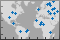## Syntax

``geoscatter(lat,lon)``
``geoscatter(lat,lon,A)``
``geoscatter(lat,lon,A,C)``
``geoscatter(___,"filled")``
``geoscatter(___,mkr)``
``geoscatter(tbl,latvar,lonvar)``
``geoscatter(tbl,latvar,lonvar,"filled")``
``geoscatter(ax,___)``
``geoscatter(___,Name,Value)``
``s = geoscatter(___)``

## Description

### Vector Data

````geoscatter(lat,lon)` creates a scatter plot with circular markers in geographic coordinates. Specify the latitude coordinates in degrees using `lat`, and specify the longitude coordinates in degrees using `lon`. If the current axes is not a geographic or map axes, or if there is no current axes, then the function creates the scatter plot in a new geographic axes.```
````geoscatter(lat,lon,A)` specifies the circle sizes in points squared. To use the same size for all the circles, specify `A` as a scalar. To plot each circle with a different size, specify `A` as a vector of the same size as `lat` and `lon`.```

example

````geoscatter(lat,lon,A,C)` specifies the circle colors. You can specify one color for all the circles, or you can vary the color. For example, you can plot all red circles by specifying `C` as `"red"`.```

example

````geoscatter(___,"filled")` fills in the circles. You can use the `"filled"` option with any of the input argument combinations in the previous syntaxes.```

example

````geoscatter(___,mkr)` specifies the marker type.```

### Table Data

example

````geoscatter(tbl,latvar,lonvar)` plots the variables `latvar` and `lonvar` from the table `tbl`. To plot one data set, specify one variable for `latvar` and one variable for `lonvar`. To plot multiple data sets, specify multiple variables for `latvar`, `lonvar`, or both. If both arguments specify multiple variables, they must specify the same number of variables. (Since R2022b) ```

example

````geoscatter(tbl,latvar,lonvar,"filled")` plots the specified variables from the table with filled circles. (Since R2022b)```

````geoscatter(ax,___)` plots into the geographic axes or map axes specified by `ax`. Specify the axes as the first argument in any of the previous syntaxes.```

example

````geoscatter(___,Name,Value)` specifies properties of the scatter plot using one or more name-value arguments. For a list of properties, see Scatter Properties.```
````s = geoscatter(___)` returns the `Scatter` object. Use `s` to set properties after creating the plot. For a full list of properties, see Scatter Properties.```

## Examples

collapse all

Specify the latitude and longitude coordinates of several locations in Boston. Create a scatter plot using filled markers.

```lat = [42.3501 42.3598 42.3626 42.3668 42.3557]; lon = [-71.0870 -71.0662 -71.0789 -71.0801 -71.0662]; geoscatter(lat,lon,"filled")```

Zoom out of the plot by adjusting the limits.

`geolimits([42.3456 42.3694],[-71.0930 -71.0536])`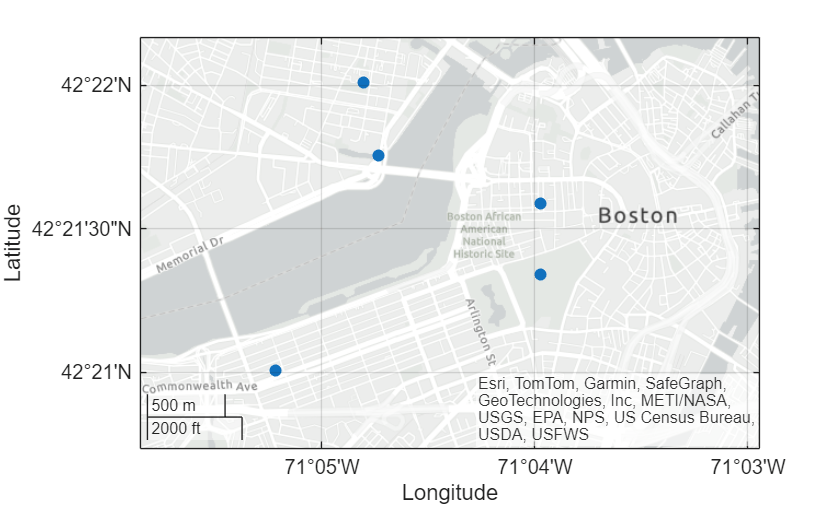Create a scatter plot using the latitude and longitude coordinates of several points along the Mississippi River. Specify the optional size and color arguments as vectors. The color values map to colors in the colormap.

```lat = [32.30 33.92 35.17 36.98 37.69 38.34]; lon = [-91.05 -91.18 -90.09 -89.11 -89.52 -90.37]; A = 100*[5 12 15 3 10 3]; C = [1 2 2 3 1 1]; geoscatter(lat,lon,A,C,"filled")```

Zoom out by adjusting the latitude and longitude limits of the plot. Then, change the basemap to a terrain basemap.

```geolimits([31.60 38.91],[-95.61 -84.27]) geobasemap grayterrain```Specify the latitude and longitude coordinates of several European cities. Create a scatter plot using magenta diamond markers.

```lat = [48.85 51.5 40.41 41.9 52.52 52.36 52.22 47.49 44.42 50.07 48.20 46.94]; lon = [2.35 -0.12 -3.70 12.49 13.40 4.90 21.01 19.04 26.10 14.43 16.37 7.44]; geoscatter(lat,lon,"m","d")```

Zoom out by adjusting the latitude and longitude limits of the plot. Then, change the basemap to a topographic basemap.

```geolimits([30 60],[-13 43]) geobasemap topographic```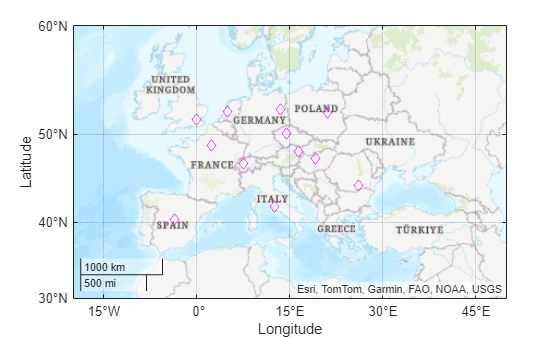Specify the latitude and longitude coordinates of several locations in Boston.

```lat = [42.3501 42.3598 42.3626 42.3668 42.3557]; lon = [-71.0870 -71.0662 -71.0789 -71.0801 -71.0662];```

Create a scatter plot and set the marker edge color, marker face color, and line width.

```geoscatter(lat,lon,70,"MarkerEdgeColor",[0.9290 0.6940 0.1250], ... "MarkerFaceColor",[0.3010 0.7450 0.9330],"LineWidth",2)```

Zoom out of the plot by adjusting the limits. Then, change the basemap.

```geolimits([42.3456 42.3694],[-71.0930 -71.0536]) geobasemap streets-dark```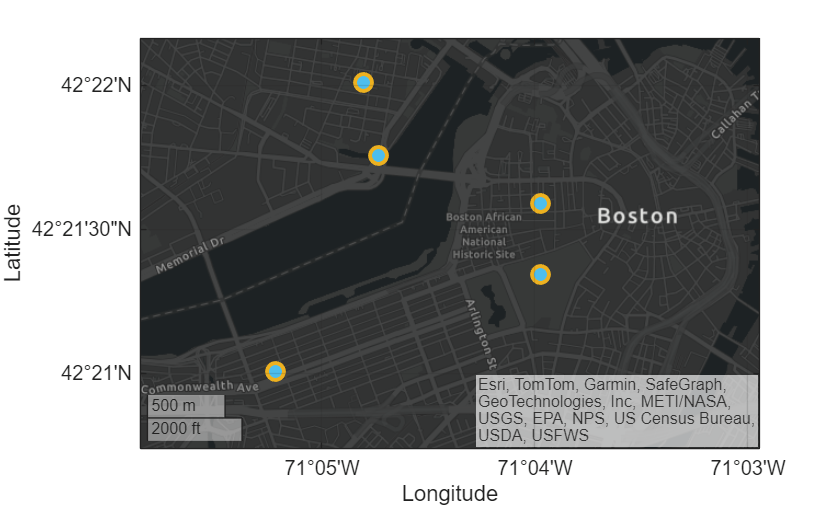Since R2022b

A convenient way to plot data from a table is to pass the table to the `geoscatter` function and specify the variables to plot.

Load a file containing county data into the workspace as a table. The table includes latitude and longitude coordinates in the table variables `Latitude` and `Longitude`, respectively.

`tbl = readtable("counties.xlsx"); `

Plot the latitude and longitude coordinates over a two-tone basemap. Return the `Scatter` object as `s`.

```s = geoscatter(tbl,"Latitude","Longitude"); geobasemap grayland```

Change the marker style and color of the plot by setting the `Marker` and `MarkerEdgeColor` properties.

```s.Marker = "*"; s.MarkerEdgeColor = "m";```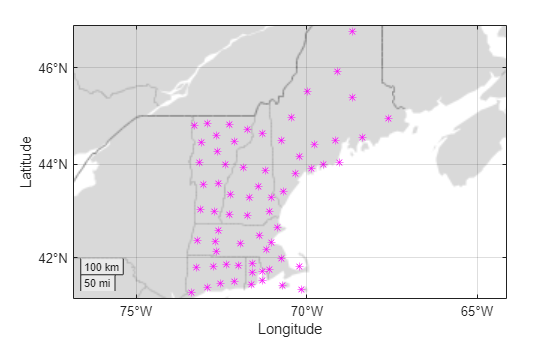Since R2022b

One way to plot data from a table and customize the colors and marker sizes is to set the `ColorVariable` and `SizeData` properties. You can set these properties as name-value arguments when you call the `geoscatter` function, or you can set them on the `Scatter` object later.

For example, load a file containing county data into the workspace as a table. The table includes latitude and longitude coordinates in the table variables `Latitude` and `Longitude`, respectively.

`tbl = readtable("counties.xlsx");`

Plot the latitude and longitude coordinates using filled markers. Return the `Scatter` object as `s`.

`s = geoscatter(tbl,"Latitude","Longitude","filled");`Change the marker sizes to `100` points by setting the `SizeData` property.

`s.SizeData = 100;`

Vary the marker colors by setting the `ColorVariable` property to a table variable. Then, add a colorbar.

```s.ColorVariable = "Population2010"; c = colorbar; c.Label.String = "County Population in 2010";```## Input Arguments

collapse all

Latitude coordinates in degrees, specified as a vector with elements in the range [–90, 90]. The vector can contain `NaN` values.

The sizes of `lat` and `lon` must match.

Example: `[43.0327 38.8921 44.0435]`

Data Types: `single` | `double`

Longitude coordinates in degrees, specified as a vector. The vector can contain `NaN` values.

The sizes of `lat` and `lon` must match.

Example: `[-107.5556 -77.0269 -72.5565]`

Data Types: `single` | `double`

Marker size, specified as a numeric scalar, numeric vector, or empty array (`[]`). The size controls the area of each marker in points squared.

• Numeric scalar — Use a uniform marker size. For example, `A = 100` creates all markers with an area of 100 points squared.

• Numeric vector — Use a different marker size for each data point. The size of `A` must match the size of `lat` and `lon`.

• Empty brackets (`[]`) — Use the default marker size of `36` points squared. Use this option when you want to specify the color input argument and use the default marker area, such as `geoscatter(lat,lon,[],c)`.

The `SizeData` property of the scatter object stores the marker sizes.

Example: `50`

Example: `[36 25 25 17 46]`

Marker color, specified as one of these options:

• RGB triplet or color name — Plot all markers with the same color.

• Three-column matrix of RGB triplets — Use different colors for each marker. Each row of the matrix specifies an RGB triplet color for the corresponding marker. The number of rows must equal the length of `lat` and `lon`.

• Vector — Use different colors for each marker and linearly map values in `C` to the current colormap. The length of `C` must equal the length of `lat` and `lon`. To change the colormap for the axes, use the `colormap` function.

An RGB triplet is a three-element row vector whose elements specify the intensities of the red, green, and blue components of the color. The intensities must be in the range `[0,1]`; for example, `[0.4 0.6 0.7]`. Alternatively, you can specify some common colors by name. This table lists the long and short color name options and the equivalent RGB triplet values.

Color NameShort NameRGB TripletAppearance
`"red"``"r"``[1 0 0]``"green"``"g"``[0 1 0]``"blue"``"b"``[0 0 1]``"cyan"` `"c"``[0 1 1]``"magenta"``"m"``[1 0 1]``"yellow"``"y"``[1 1 0]``"black"``"k"``[0 0 0]``"white"``"w"``[1 1 1]`When you specify marker colors, the `geoscatter` function sets the `MarkerFaceColor` property of the `Scatter` object to `"flat"` and stores the marker colors in the `CData` property.

Example: `"green"`

Example: `"g"`

Example: `[0 1 0]`

Marker symbol, specified as one of these values.

MarkerDescriptionResulting Marker
`"o"`Circle`"+"`Plus sign`"*"`Asterisk`"."`Point`"x"`Cross`"_"`Horizontal line`"|"`Vertical line`"square"`Square`"diamond"`Diamond`"^"`Upward-pointing triangle`"v"`Downward-pointing triangle`">"`Right-pointing triangle`"<"`Left-pointing triangle`"pentagram"`Pentagram`"hexagram"`HexagramOption to fill the interior of the markers, specified as `"filled"`. Use this option with markers that have a face, for example, `"o"` or `"square"`. This option does not display markers that do not have a face and contain only edges, such as `"+"` and `"*"`.

The `"filled"` option sets the `MarkerFaceColor` property of the `Scatter` object to `"flat"` and the `MarkerEdgeColor` property to `"none"`. As a result, this option displays the marker faces and does not display the edges.

Source table containing the data to plot, specified as a table or a timetable.

Table variables containing the latitude coordinates, specified using one of the indexing schemes from the table.

Indexing SchemeExamples

Variable names:

• `"A"` or `'A'` — A variable called `A`

• `["A","B"]` or `{'A','B'}` — Two variables called `A` and `B`

• `"Var"+digitsPattern(1)` — Variables named `"Var"` followed by a single digit

Variable index:

• An index number that refers to the location of a variable in the table.

• A vector of numbers.

• A logical vector. Typically, this vector is the same length as the number of variables, but you can omit trailing `0` or `false` values.

• `3` — The third variable from the table

• `[2 3]` — The second and third variables from the table

• `[false false true]` — The third variable

Variable type:

• `vartype("categorical")` — All the variables containing categorical values

Regardless of the variable name, the axis label on the plot is always `Latitude`.

The variables you specify must contain numeric data of type `single` or `double`. The data must be in the range (–90, 90).

If `latvar` and `lonvar` both specify multiple variables, the number of variables must be the same.

Example: `geoscatter(tbl,["lat1","lat2"],"lon")` specifies the table variables named `lat1` and `lat2` for the latitude coordinates.

Example: `geoscatter(tbl,2,"lon")` specifies the second variable for the latitude coordinates.

Example: `geoscatter(tbl,vartype("numeric"),"lon")` specifies all numeric variables for the latitude coordinates.

Table variables containing the longitude coordinates, specified using one of the indexing schemes from the table.

Indexing SchemeExamples

Variable names:

• `"A"` or `'A'` — A variable called `A`

• `["A","B"]` or `{'A','B'}` — Two variables called `A` and `B`

• `"Var"+digitsPattern(1)` — Variables named `"Var"` followed by a single digit

Variable index:

• An index number that refers to the location of a variable in the table.

• A vector of numbers.

• A logical vector. Typically, this vector is the same length as the number of variables, but you can omit trailing `0` or `false` values.

• `3` — The third variable from the table

• `[2 3]` — The second and third variables from the table

• `[false false true]` — The third variable

Variable type:

• `vartype("categorical")` — All the variables containing categorical values

Regardless of the variable name, the axis label on the plot is always `Longitude`.

The variables you specify must contain numeric data of type `single` or `double`.

If `latvar` and `lonvar` both specify multiple variables, the number of variables must be the same.

Example: `geoscatter(tbl,"lat",["lon1","lon2"])` specifies the table variables named `lon1` and `lon2` for the longitude coordinates.

Example: `geoscatter(tbl,"lat",2)` specifies the second variable for the longitude coordinates.

Example: `geoscatter(tbl,"lat",vartype("numeric"))` specifies all numeric variables for the longitude coordinates.

Target axes, specified as a `GeographicAxes` object1 or `MapAxes` (Mapping Toolbox™) object.

If you do not specify this argument, then the `geoscatter` function plots into the current axes, provided that the current axes is a geographic axes or map axes object.

### Name-Value Arguments

Specify optional pairs of arguments as `Name1=Value1,...,NameN=ValueN`, where `Name` is the argument name and `Value` is the corresponding value. Name-value arguments must appear after other arguments, but the order of the pairs does not matter.

Example: `geoscatter(lat,lon,"filled",MarkerFaceAlpha=0.5)` creates filled, semi-transparent markers.

Before R2021a, use commas to separate each name and value, and enclose `Name` in quotes.

Example: `geoscatter(lat,lon,"filled","MarkerFaceAlpha",0.5)` creates filled, semi-transparent markers.

The scatter object properties listed here are only a subset. For a complete list, see Scatter Properties.

Marker outline color, specified `"flat"`, an RGB triplet, a hexadecimal color code, a color name, or a short name. The default value of `"flat"` uses colors from the `CData` property.

For a custom color, specify an RGB triplet or a hexadecimal color code.

• An RGB triplet is a three-element row vector whose elements specify the intensities of the red, green, and blue components of the color. The intensities must be in the range `[0,1]`, for example, ```[0.4 0.6 0.7]```.

• A hexadecimal color code is a string scalar or character vector that starts with a hash symbol (`#`) followed by three or six hexadecimal digits, which can range from `0` to `F`. The values are not case sensitive. Therefore, the color codes `"#FF8800"`, `"#ff8800"`, `"#F80"`, and `"#f80"` are equivalent.

Alternatively, you can specify some common colors by name. This table lists the named color options, the equivalent RGB triplets, and hexadecimal color codes.

Color NameShort NameRGB TripletHexadecimal Color CodeAppearance
`"red"``"r"``[1 0 0]``"#FF0000"``"green"``"g"``[0 1 0]``"#00FF00"``"blue"``"b"``[0 0 1]``"#0000FF"``"cyan"` `"c"``[0 1 1]``"#00FFFF"``"magenta"``"m"``[1 0 1]``"#FF00FF"``"yellow"``"y"``[1 1 0]``"#FFFF00"``"black"``"k"``[0 0 0]``"#000000"``"white"``"w"``[1 1 1]``"#FFFFFF"``"none"`Not applicableNot applicableNot applicableNo color

Here are the RGB triplets and hexadecimal color codes for the default colors MATLAB® uses in many types of plots.

`[0 0.4470 0.7410]``"#0072BD"``[0.8500 0.3250 0.0980]``"#D95319"``[0.9290 0.6940 0.1250]``"#EDB120"``[0.4940 0.1840 0.5560]``"#7E2F8E"``[0.4660 0.6740 0.1880]``"#77AC30"``[0.3010 0.7450 0.9330]``"#4DBEEE"``[0.6350 0.0780 0.1840]``"#A2142F"`Example: `[0.5 0.5 0.5]`

Example: `"blue"`

Example: `"#D2F9A7"`

Marker fill color, specified as `"flat"`, `"auto"`, an RGB triplet, a hexadecimal color code, a color name, or a short name. The `"flat"` option uses the `CData` values. The `"auto"` option uses the same color as the `Color` property for the axes.

For a custom color, specify an RGB triplet or a hexadecimal color code.

• An RGB triplet is a three-element row vector whose elements specify the intensities of the red, green, and blue components of the color. The intensities must be in the range `[0,1]`, for example, ```[0.4 0.6 0.7]```.

• A hexadecimal color code is a string scalar or character vector that starts with a hash symbol (`#`) followed by three or six hexadecimal digits, which can range from `0` to `F`. The values are not case sensitive. Therefore, the color codes `"#FF8800"`, `"#ff8800"`, `"#F80"`, and `"#f80"` are equivalent.

Alternatively, you can specify some common colors by name. This table lists the named color options, the equivalent RGB triplets, and hexadecimal color codes.

Color NameShort NameRGB TripletHexadecimal Color CodeAppearance
`"red"``"r"``[1 0 0]``"#FF0000"``"green"``"g"``[0 1 0]``"#00FF00"``"blue"``"b"``[0 0 1]``"#0000FF"``"cyan"` `"c"``[0 1 1]``"#00FFFF"``"magenta"``"m"``[1 0 1]``"#FF00FF"``"yellow"``"y"``[1 1 0]``"#FFFF00"``"black"``"k"``[0 0 0]``"#000000"``"white"``"w"``[1 1 1]``"#FFFFFF"``"none"`Not applicableNot applicableNot applicableNo color

Here are the RGB triplets and hexadecimal color codes for the default colors MATLAB uses in many types of plots.

`[0 0.4470 0.7410]``"#0072BD"``[0.8500 0.3250 0.0980]``"#D95319"``[0.9290 0.6940 0.1250]``"#EDB120"``[0.4940 0.1840 0.5560]``"#7E2F8E"``[0.4660 0.6740 0.1880]``"#77AC30"``[0.3010 0.7450 0.9330]``"#4DBEEE"``[0.6350 0.0780 0.1840]``"#A2142F"`Example: `[0.3 0.2 0.1]`

Example: `"green"`

Example: `"#D2F9A7"`

Width of marker edge, specified as a positive value in point units.

Example: `0.75`

Table variable containing the color data, specified as a variable index into the source table.

#### Specifying the Table Index

Use any of the following indexing schemes to specify the desired variable.

Indexing SchemeExamples

Variable name:

• A string scalar or character vector.

• A `pattern` object. The pattern object must refer to only one variable.

• `"A"` or `'A'` — A variable called `A`

• `"Var"+digitsPattern(1)` — The variable with the name `"Var"` followed by a single digit

Variable index:

• An index number that refers to the location of a variable in the table.

• A logical vector. Typically, this vector is the same length as the number of variables, but you can omit trailing `0` or `false` values.

• `3` — The third variable from the table

• `[false false true]` — The third variable

Variable type:

• A `vartype` subscript that selects a table variable of a specified type. The subscript must refer to only one variable.

• `vartype("double")` — The variable containing double values

#### Specifying Color Data

Specifying the `ColorVariable` property controls the colors of the markers. The data in the variable controls the marker fill color when the `MarkerFaceColor` property is set to `"flat"`. The data can also control the marker outline color, when the `MarkerEdgeColor` is set to `"flat"`.

The table variable you specify can contain values of any numeric type. The values can be in either of the following forms:

• A column of numbers that linearly map into the current colormap.

• A three-column array of RGB triplets. RGB triplets are three-element vectors whose values specify the intensities of the red, green, and blue components of specific colors. The intensities must be in the range `[0,1]`. For example, `[0.5 0.7 1]` specifies a shade of light blue.

When you set the `ColorVariable` property, MATLAB updates the `CData` property.

## Output Arguments

collapse all

Geographic scatter plot, returned as a `Scatter` object. Use `s` to access and modify properties of the geographic scatter plot after it has been created.

## Tips

• When you plot on geographic axes, the `geoscatter` function assumes that coordinates are referenced to the WGS84 coordinate reference system. If you plot using coordinates that are referenced to a different coordinate reference system, then the coordinates may appear misaligned.

## Version History

Introduced in R2018b

expand all